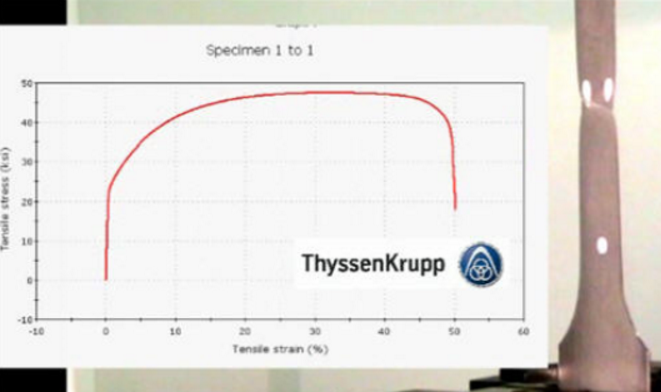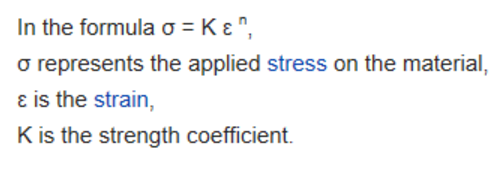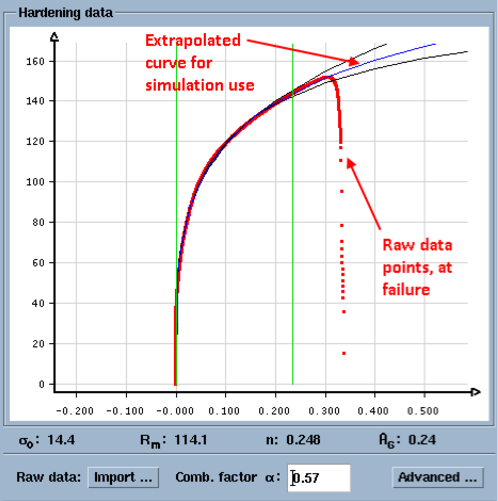# Evaluation of Sheet Metal Formability Through Tensile Tests

Sheet metal forming simulation is almost meaningless, without accurate material data. The old saying “garbage in, garbage out” rings true in this regard.

To correctly and accurately capture the mechanical properties of material for use in simulation, a tensile test is performed. The testing procedure is further explained in this article:

Stamping Simulation: Material Tensile Testing Explained### What can we learn from the tensile test results and what are the usual outputs from a tensile test?

Most commonly discussed and understood are these test results, which are provided at the conclusion of any uniaxial tensile test:

• Yield Strength (YS) MPa or ksi
• Ultimate Tensile Strength (UTS) MPa or ksi
• Elongation %

But much less discussion and focus is put on the “n value” or the shape of the stress-strain data curve, both of which are outputs from a tensile test. The shape of the data curve and the “n value” tells more about the formability of the material than the other recorded values.

Although the “n value” is the exponent which provides the shape of the stress-strain curve, the mathematical fitting of an equation to the curve with a single exponent may not best represent the data curve. For this reason, much more advanced mathematical methods may be used to more correctly represent the true shape of the data points collected as part of the tensile test.Furthermore, the raw data points can’t be collect after the point of failure but simulation software requires that the stress-strain curve be extrapolate past the failure point (UTS) such that FEA values may continue to be calculated in the mesh. Simulation software calculates values as though no failure occurs, but then applies sheet metal failure theory or a Forming Limit Diagram (FLD) to determine which elements in the mesh have failed or are close to fail.While the YS and UTS will tell us a material’s strength it does little to tell us how the material will form. This is where the “n value” can help. Very broadly speaking, the value of n can be related to the formability of a given material. For example, if n=0.23 or greater, the steel may be considered to be a deep draw material OR if n=0.15 or less, this may indicate that the material is difficult to form and may not be suitable for product designs with deep features.

As a side note, material hardness (the surface hardness of a given steel) has almost no relation to the “n value” or the material’s formability. It is commonly mis-stated that a “hard” material will not form easily…only a tensile test and subsequent simulation can determine the real outcome.